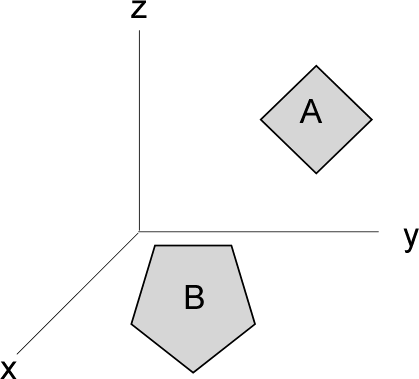Contents

F3D_polyProcedure f3d_poly(NA,NB,xA[1..NA],yA[1..NA],zA[1..NA],xB[1..NB],yB[1..NB],zB[1..NB]:F_AB,F_BA,areaA,areaB)

The F3D_poly procedure returns the view factors between two polygons as well as their areas provided the following conditions are met:
1) polygons are planar (all vertices lie in the same plane)
2) polygons are simple (no self-intersecting polygons)
3) polygons are convex (in theory, concave polygons should work, but this remains untested)

Author: Jacob Kerkhoff, University of Wisconsin-Madison, Solar Energy Laboratory

Analytical solution derivation:
Narayanaswamy, Arvind. "An analytic expression for radiation view factor between two arbitrarily oriented planar polygons." International Journal of Heat and Mass Transfer 91 (2015): 841-847.

Inputs:
NA = number of vertices for polygon A
NB = number of vertices for polygon B

xA[1..NA] = array containing the x-coordinates of polygon A vertices (m or ft)
yA[1..NA] = array containing the y-coordinates of polygon A vertices (m or ft)
zA[1..NA] = array containing the z-coordinates of polygon A vertices (m or ft)

xB[1..NA] = array containing the x-coordinates of polygon B vertices (m or ft)
yB[1..NA] = array containing the y-coordinates of polygon B vertices (m or ft)
zB[1..NA] = array containing the z-coordinates of polygon B vertices (m or ft)

Outputs:
F_AB = view factor from polygon A to polygon B
F_BA = view factor from polygon B to polygon A
areaA = area of polygon A (m^2 or ft^2)
areaB = area of polygon B (m^2 or ft^2)

Example:
//Test case C, square and a pentagon, view factor should be F_AB = 0.1541243
NA=5
xA[1..5]=[0,0,0.5,1,1]
yA[1..5]=[0,1,2,1,0]
zA[1..5]=[0,0,0,0,0]
NB=4
xB[1..4]=[0,0,0,0]
yB[1..4]=[0,1,1,0]
zB[1..4]=[0,0,1,1]
Call  f3d_poly(NA,NB,xA[1..NA],yA[1..NA],zA[1..NA],xB[1..NB],yB[1..NB],zB[1..NB]:F_AB,F_BA,areaA,areaB)
{F_AB = 0.1541, F_BA = 0.2312, areaA = 1.5 [m^2], areaB = 1 [m^2]}

View Factor Index